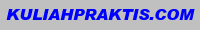## Selamat Datang di Elearning Faqih.Net

Blog Elearning Faqih.Net adalah blog media e-learning. Siapapun bebas mengunjungi blog ini untuk belajar, berbagi ilmu pengetahuan dan informasi, serta saling bertukar pikiran dan saling membantu dalam proses belajar. Ayo...Belajar bersama di Elearning.Faqih.Net

## Sabtu, 12 Desember 2009

### Tweet Kuliah Mekanika Teknik TI - Keseimbangan Partikel (2D)

KULIAH II
MEKANIKA TEKNIK TI
KESEIMBANGAN PARTIKEL (2D)
OLEH:
ALIEF WIKARTA, ST
JURUSAN TEKNIK MESIN,
FTI – ITS SURABAYA, 2007
Equilibrium of a Particle (2-D)
Today’s Objectives:
Students will be able to :
a) Draw a free body diagram (FBD), and,
b) Apply equations of equilibrium to solve a 2-D problem.
For a given cable strength, what is the maximum weight that can be lifted ?
Free Body Diagram (FBD) (2-D)
Equations of Equilibrium (2-D)
Since particle A is in equilibrium, the net force at A is zero.
So FAB + FAD + FAC = 0
or   F = 0
Or, written in a scalar form,
These are two scalar equations of equilibrium (EofE).  They can be used to solve for up to two unknowns.
• Fx = 0  and Fy = 0

In general, for a particle in equilibrium, F = 0   or
Fx Fy j   =   0   =  0 +  0 j    (A vector equation)
EXAMPLE
Write the scalar EofE:

+ Fx = TB cos 30º    T =   0
+ Fy  =  TB sin 30º    2.452 kN = 0

Solving the second equation gives: TB = 4.90 kN
From the first equation, we get: TD = 4.25 kN
Note : Engine mass = 250 Kg

FBD at  A
Problem Solving (2-D)

Given: The car is towed at constant speed by the 600 N force and the angle is 25°.
Find:    The forces in the ropes AB and AC.
Plan:
1. Draw a FBD for point A.
2. Apply the EofE to solve for the forces in ropes AB and AC.
F = 600 N

θ = 25o
Problem Solving (2-D)

F = 600 N
θ = 25o
30°
25°
600 N
FAB

FAC

A
FBD at point A

Applying the scalar EofE at A, we get;
+ Fx = FAC cos 30° – FAB cos 25° = 0
+ Fy = -FAC sin 30° – FAB sin 25° + 600  = 0
Solving the above equations, we get;
FAB = 634 N
FAC = 664 N
In a ship-unloading operation, a 15.6 kN automobile is supported by a cable. A rope is tied to the cable and pulled to center the automobile over its intended position. What is the tension in the rope?
SOLUTION:
• Construct a free-body diagram for the particle at the junction of the rope and cable.

• Determine the unknown force magnitudes.

Example
EXAMPLE
Given: Sack A weighs 20 N. and geometry is as shown.
Find: Forces in the cables and weight of sack B.
Plan:
1. Draw a FBD for Point E.
2. Apply EofE at Point E to solve for the unknowns (TEG & TEC).
3. Repeat this process at C.
EXAMPLE (continued)
The scalar EofE are:
+    Fx = TEG  sin 30º –  TEC cos 45º = 0
+     Fy = TEG cos 30º –  TEC sin 45º  – 20 N  = 0

Solving these two simultaneous equations for the two unknowns yields:
TEC = 38.6 N
TEG = 54.6 N
A FBD at E should look like the one to the left.  Note the assumed directions for the two cable tensions.
EXAMPLE (continued)
Fx = 38.64 cos 45  – (4/5) TCD  =  0
Fy  = (3/5) TCD +  38.64 sin 45  –  WB  =  0
Solving the first equation and then the second yields
TCD = 34.2 N     and    WB = 47.8 N .
The scalar EofE are:

Now move on to ring C.   A FBD for C should look like the one to the left.
1) When a particle is in equilibrium, the sum of forces acting on it equals ___ . (Choose the most appropriate answer)
A) a constant B) a positive number C) zero
D) a negative number E) an integer.
2) For a frictionless pulley and cable, tensions in the cable (T1 and T2) are related as _____ .
A) T1 > T2
B) T1 = T2
C) T1 < T2
D) T1 = T2 sin
ATTENTION QUIZ
F2

20 N
F1

C
50°
3. Using this FBD of Point C, the sum of forces in the x-direction ( FX) is ___ .   Use a sign convention of +  .
A) F2 sin 50°  – 20  = 0
B) F2 cos 50°  – 20  = 0
C) F2 sin 50°  – F1   = 0
D) F2 cos 50° + 20  = 0
Jika b = 4 m,
tentukan harga P dan jarak a
SOAL TANTANGAN
TERIMA KASIH

## Welcome

Pasang Banner KuliahPraktis.com di Blogmu